# An Elastic-plastic Constitutive Model of Concrete Based on Thermodynamic Principles and Its Application in Arch Dam Design

An Elastic-plastic Constitutive Model of Concrete Based on Thermodynamic Principles and Its Application in Arch Dam Design
Chongqing Water Resources and electric engineering college, Chongqing 402160, China

Corresponding Author Email:
xj_cqsdzy@163.com
Page:
267-272
|
DOI:
https://doi.org/10.18280/ijht.370132
19 July 2018
|
Accepted:
9 October 2018
|
Published:
31 March 2019
| Citation

OPEN ACCESS

Abstract:

The safety of arch dams is greatly affected by the nonlinearity of the concrete. This paper introduces the basic theories on concrete constitutive models, analyzes the incremental energy dissipation function of concrete in the plastic process, and derives an elastic-plastic constitutive model for concrete based on thermodynamic principles. The proposed model was applied to examine the dynamic nonlinear seismic responses of an actual arch dam, and compared with several other models. Besides, the effect of strain rate on the concrete performance was also discussed in details. The research findings provide a reference for the safety evaluation of arch dam design.

Keywords:

concrete, constitutive model, thermodynamic principals, elastic-plastic, dam safety

1. Instruction

The dam safety is an important guarantee for the normal production and living of downstream residents. The safety issues must be fully considered in the design phase, and carefully evaluated after construction to see if it satisfies the design criteria. Traditionally, the mechanical safety of dams is mainly analyzed in terms of the linear elastic response. However, the linear elastic analysis cannot capture the exact structural response, especially in seismic activities. In recent years, the research focus has gradually shifted to the nonlinear seismic response.

An arch dam is a concrete dam that is curved upstream in plan. The safety of such a dam lies heavily on the damage mechanism of concrete. The concrete properties can be examined by several dynamic models [1-7]. However, it is necessary to construct a rational constitutive model of concrete with few assumptions and clear physical meaning, before exploring the nonlinear responses of arch dams. The constitutive model should reflect the mechanical properties of concrete in all deformation phases, and depict the progress failure from strain softening, strength reduction to stiffness reduction, making it possible to identify the nonlinear features of concrete. The model should also conform to the general principle of energy and carry the nonlinear features of concrete.

As a nonlinear factor, the rate dependency of concrete must be considered in dam safety analysis. The theory of metal plasticity has long been applied to nonlinear analysis of concrete, creating many plastic and viscoplastic models. Nonetheless, many problems have arisen in these models, due to the difference between concrete and metals. Thus, this theory must be further modified before being applied to concrete. In addition, the redistribution of structural stress should not be included in dam safety analysis, aiming to reduce the measurement error of safety behavior of the structure.

In light of the above, this paper develops a proper constitutive model of concrete based on thermodynamic principles, and adopts the model to analyze the static and dynamic responses of arch dams, laying the basis for dam safety evaluation.

2. Literature Review

Concrete is an important material in civil engineering.  Traditionally, the mechanical analysis on concrete mainly focuses on the linear elastic response of the structure, owing to the limited computing power and insufficient understanding of the material. As concrete structures become increasingly complex, it is of great significance to analyze the nonlinear response of the structure in an accurate manner. However, the popularity of nonlinear analysis has been restricted by many factors, e.g. the concrete properties in confined structure cannot be simulated directly [8, 9].

Recent years saw the emergence of various constitutive models for concrete, such as nonlinear elastic model and plastic model. Among them, the nonlinear elastic model is often fitted by empirical formulas, and employed to discuss the nonlinear compressive deformation of concrete under monotonic loading. This model is hailed for the simple computation and stable algorithm. Nevertheless, the model cannot reveal the complex performance of concrete, as it requires a large range of stress-strain data. Some of the nonlinear elastic constitutive models use the variable secant modulus to depict the full-scale stress-strain relationship [10-11], and some illustrate the incremental stress-strain relationship [12-13].

The plastic constitutive model is grounded on the plasticity theory, which describes the relationship between stress increment and strain increment of a material in plastic state. This theory is one of the instruments on the macro stress-strain features of concrete, alongside with damage theory and endochronic theory. Based on this theory, the plastic constitutive models have been created to illustrate the loading path, hardening and softening of concrete [14-18]. However, the plasticity theory and its related models cannot accurately simulate the macroscale decline of concrete integrity and change of concrete performance, which come from the microcrack propagation and penetration under external load.

To overcome the defect, the damage theory  has been introduced to the analysis on concrete properties. In the damage constitutive models of concrete, the damage variable and damage evolution, the two basic factors of damage mechanics, are introduced into the stress-strain relationship for accurate depiction of concrete deterioration. The typical damage constitutive models include Loland model , Mazars model , piecewise linear damage model  and piecewise curve damage model .

The endochronic theory was conceptualized to overcome the difficulties of the plastic theory in experiment and numerical calculation using the yield surface. In this theory, the yield surface is scrapped to avoid the said difficulties. The endochronic constitutive model was initially applied to metals, and introduced to concrete by Bazant et al. . This model can describe the exact constitutive phenomena of concrete, as it is thermodynamically irreversible and free from the constraint of the yield surface. Nonetheless, the endochronic model is too complex to be applied widely in practice.

3. Mathematical Derivation

This section explains the mathematical derivation of a concrete constitutive model based on thermodynamic principles.

According to the first and second laws of thermodynamics, a material under isothermal deformation obeys the following energy relationship:

$dW=dF+dD, dD≥0$ (1)

where $dW=σ:dε$ is the incremental stress; dF is the incremental free energy; dD is the incremental energy dissipation. The free energy is a function of independent observable state variables like the total strain $ε$ and the plastic strain $ε^P$. Hence, the incremental free energy can be defined as:

$dF=∂F/∂ε:dε+∂F/(∂ ε^P ):d ε^P$ (2)

The energy dissipation depends both on state variables and the incremental plastic strain. However, it has nothing to do with the total incremental strain (otherwise, a purely elastic deformation will dissipate energy). For a non-elastic-plastic material, the incremental energy dissipation dD can be described by the first-order homogeneous function of incremental plastic strain:

$dD=(∂(dD))/(∂(dε^P)):dε^P$(3)

Substituting the expressions of dH and dD into Equation (1), we have:

$σ:dε=∂F/∂ε:dε+(∂F/(∂ ε^P )+∂(dD)/∂(dε^P ) ): dε^P$ (4)

Eliminating dH and dD, we have:

$σ=∂F/∂ε$ (5)

Meanwhile, the dissipative stress, i.e. the generalized stress in dissipative stress space, can be defined as:

$χ=∂F/(∂ ε^P )=-(∂(dD))/(∂(dε^P))$ (6)

The value of the dissipative stress can be derived either from free energy function or from dissipative function. Eliminating dε^P in Equation (6), the yield function f(χ)=0 can be obtained for the dissipative stress space.

For an elastic-plastic material, the free energy can be expressed as the sum of the elastic strain and the plastic strain:

$F=F_1 (ε^e )+F_1 (ε^P )$ (7)

The elastic part of the above equation can be expressed as:

$dW^e=σ:dε^e=dF_1=(∂F_1 (ε^e))/(∂ε^e ):dε^e$ (8)

Thus, the elastic constitutive relation can be obtained as:

$σ=(∂F_1 (ε^e))/(∂ε^e )$ (9)

The plastic part of the Equation (7):

$dW^P=σ:dε^P=dF_2+dD=(∂F_2 (ε^P))/(∂ε^P ): dε^P+(∂(dD))/(∂(dε^P)): dε^P$ (10)

Thus, the plastic constitutive relation can be obtained as:

$σ=(∂F_2 (ε^P))/(∂ε^P )+(∂(dD))/(∂(dε^P))=ρ+χ$ (11)

where $χ$ is the dissipation stress; $ρ$ is the shift stress.

4. Constitutive Model Construction

4.1 Yield and failure surfaces in dissipative stress space

The plastic deformation of concrete is irreversible and energy-dissipating. For simplicity, the concrete deformation was divided into volume deformation and shear deformation. For the two types of deformation, the stresses were denoted as hydrostatic pressure p and shear stress q, respectively, and the strains were defined as volumetric strain $ε_V$ and shear strain $ε_S$, respectively. It is assumed that the plastic volumetric strain is independent of the plastic shear strain, and correspond to different dissipation processes.

The incremental energy dissipation can be defined as:

$dD=√(((Adε_V)^2+(Bdε_S)^2 ))$ (12)

where A and B are dimensions of stress. The two parameters can be viewed as functions of $p$, $q$, $p_c$ and $p_t$. The simplest linear form of A and B can be written as:

$A=a_1 p+a_2 q+a_3 (p_t-p_c)$ (13)

$B=b_1 p+b_2 q+b_3 (p_t-p_c)$ (14)

where $a_1$, $a_2$, $a_3$ and $b_1$, $b_2$, $b_3$ are dissipation parameters.

According to the dissipation function, the volumetric dissipative stress and shear dissipative stress can be respectively obtained as:

$π=(∂(dD))/(∂(dε_V^P))=A^2/dD dε_V^P$ (15)

$τ=(∂(dD))/(∂(dε_S^P))=B^2/dD dε_S^P$ (16)

Eliminating the incremental plastic volumetric strain $ε_V$ and incremental plastic shear strain $ε_S$, the yield condition in dissipative stress space can be expressed as:

$π^2/A^2 +τ^2/B^2 =1$ (17)

Obviously, the yield function and failure function in the dissipative stress space are a family of ellipses on the $π-τ$ plane. The shape of yield function in dissipative stress space is described in Figure 1, where O is the origin of the circle, and the axes π and τ are the long and short axes, respectively.

## 1.jpgFigure 1. The shape of yield function in dissipative stress space

Let $ξ$ and $η$ be the migration stresses in the direction of volume stress and shear stress, respectively. During the plastic deformation, the value of $η$ equals zero, for the trajectory of yield surface in plane $π$ always centers on the hydrostatic pressure axis. In other words, the yield surface and failure surface only change horizontally due to the zero migration stress, when being mapped from the dissipative stress space to real stress space. The relationship between real stress and dissipative stress can be defined as:

$p= ξ+π$ (18)

$q=τ$ (19)

The shape of the yield function in real stress space is shown in Figure 2.

## 2.jpg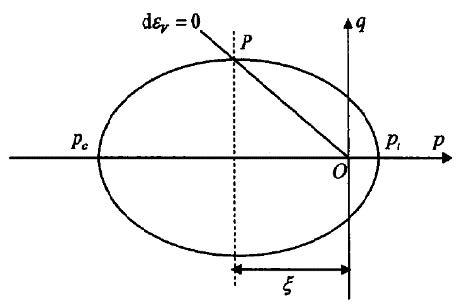Figure 2. The shape of yield function in real stress space

The intersection point ($0, τ$) of $τ$ axis and yield surface in dissipative stress space was mapped to ($ξ, τ$) in real stress space. Thus, the migration stress in the $τ$ direction equals the volumetric stress at the intersection of the yield surface and the line with zero plastic volumetric strain in the real stress space.

4.2 Stress-strain relationship

The stress-strain relationship of the elastic part can be obtained from the generalized Hook’s law , while that of the plastic part can be deduced in the following manner:

The strain increment can be expressed as the sum of elastic and plastic parts.

$dε=dε^e+dε^P$  (20)

According to the flow theory, we have:

$dε^P=dλm$ (21)

From the generalized Hooke’s law, the incremental elastic strain can be expressed as:

$dε^e=D^(-1) dσ$  (22)

Hence, Equation (20) can be rewritten as:

$dε=D^(-1) dσ+dλm$ (23)

In the dissipative stress space, the yield function always takes an elliptical shape, however the variation in the parameters. For the convenience of plotting, the yield function can be converted into a parametric equation:

$π=A cos⁡ω,τ=B sin⁡ω$ (24)

where $ω$ is the auxiliary angle.

Substituting stress dimensions and migration stress into Equation (24), we have:

$\left\{ \begin{matrix} p-1/2\text{ }\gamma \left( {{p}_{t}}-{{p}_{c}}\text{ } \right)=\left( {{a}_{1}}\text{ }p+{{a}_{2}}\text{ }q+{{a}_{3}}\left( {{p}_{t}}-{{p}_{c}}\text{ } \right) \right)cos\omega \\ q=\left( {{b}_{1}}\text{ }p+{{b}_{2}}\text{ }q+{{b}_{3}}\text{ }\left( {{p}_{t}}-{{p}_{c}}\text{ } \right) \right)sin\omega \\\end{matrix} \right.$  (25)

The parametric equation of the yield function in real stress space can be solved as:

$\left\{ \begin{matrix} p=\left( \left( 1-{{b}_{2}}~sin\omega \right)\left( 1/2\text{ }\gamma +{{a}_{3}}~\text{ }cos\omega \right)+{{a}_{2}}\text{ }{{b}_{3}}~\text{ }sin\omega ~\text{ }cos\omega \right)\left( {{p}_{t}}-{{p}_{c}} \right)/\Delta \\ q=\left( {{b}_{1}}~sin\omega \text{ }\left( 1/2\text{ }\gamma +{{a}_{3}}~cos\omega \right)+{{b}_{3}}~sin\omega ~\text{ }\left( 1-{{a}_{1}}\text{ }cos\omega \right) \right)\left( {{p}_{t}}-{{p}_{c}} \right)/\Delta \\\end{matrix} \right.$ (26)

where,

$Δ=(1-b_2 sin⁡ω )(1-a_1 cos⁡ω )-a_2 b_1 sin⁡ω cos⁡ω$.

After the mapping to the real stress space, the shape of the yield function is controlled by the parameters of each model. The stress points on the yield surface have a complex mapping relationship between the two stress spaces. Many anomalies may occur if the model parameters are selected randomly. Thus, the range of these parameters should be determined.

The yield function in real stress space was plotted by Equation (26). An abnormal shape of the equation is presented in Figure 3. The anomality occurs at $ω∈[π⁄2,3π⁄2]$. For avoid the anomality, the yield function should satisfy:

$p<0,q≥0$  if $ω∈[π⁄2,π]$

$p<0,q≤0$ if $ω∈[π,3π⁄2]$ (27)

## 3.jpg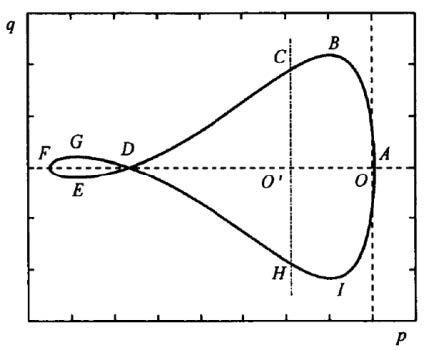Figure 3. Abnormal yield surface in real stress space

Next, a quadrant analysis was performed to analyze the influence of other parameters on the shape of yield function. The extreme values of yield surfaces are displayed in Figure 4, where  O' is the origin of dissipative stress space, also the origin of the ω  parameter coordinate system.

## 4.jpg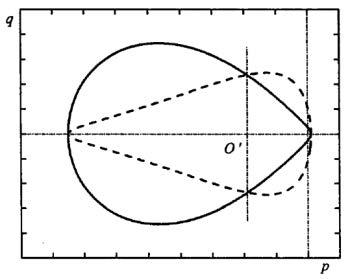Figure 4. Extreme value of yield surfaces

The yield function takes the shape of curve ABC when ω∈[0,π⁄2], that of curve CDEF when $ω∈[π⁄2,π]$, that of curve FGDH when $ω∈[ π,3π⁄2]$, and that of curve HIA when $ω∈[3π⁄2,2π]$.

The constitutive model has five independent parameters. To disclose the effect of each parameter on the yield function, four parameter combinations were designed (Table 1). The values of parameters $p_t$ and $p_c$ remained constant at 0.2 and -15, respectively. The yield function shapes of these combinations in the real stress space were drawn, and compared with each other.

Table 1. Parameter combinations and corresponding yield functions in real stress space

 Parameters Combination 1 Combination 2 Combination 3 Combination 4 γ -0.5, -1, -1.5 -1 -1 -1 b1 -0.5 -0.3, -0.5, -0.7 -0.5 -0.3, -0.5, -0.7 b3 0.25 0.25 0.15, 0.25, 0.35 0.2, 0.25, 0.3 Yield function shape Figure 5 Figure 6 Figure 7 Figure 8

## 5.jpg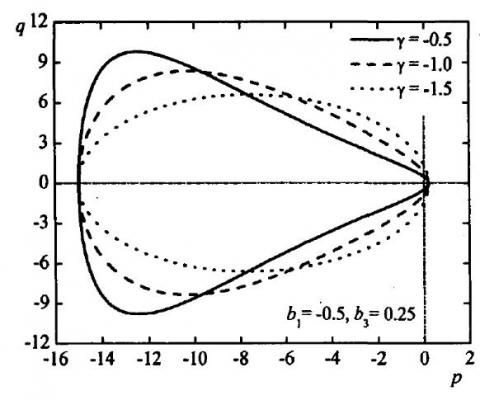Figure 5. The shape of the yield function for combination 1

## 6.jpg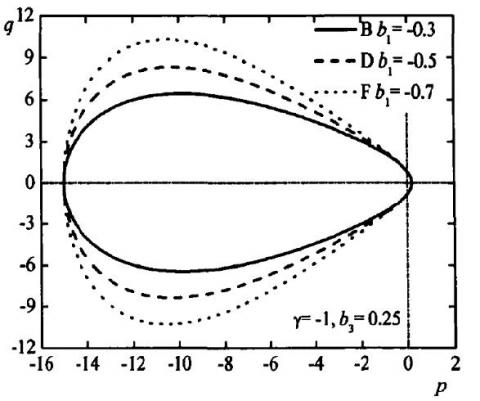Figure 6. The shape of the yield function for combination 2

## 7.jpg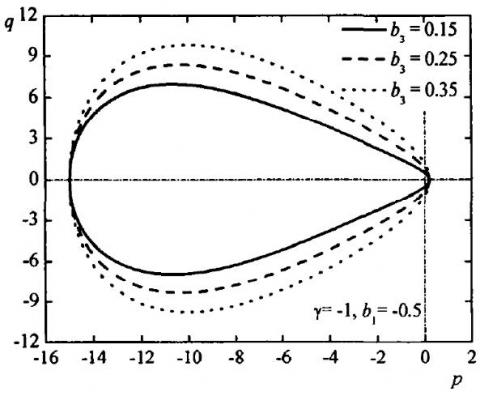Figure 7. The shape of the yield function for combination 3

## 8.jpg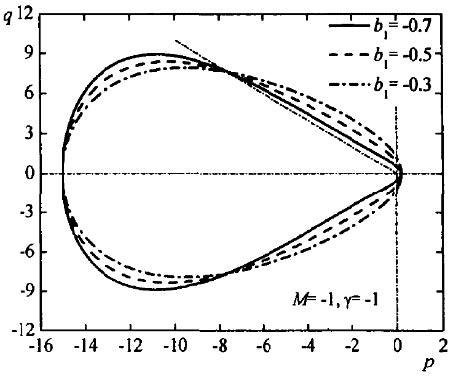Figure 8. The shape of the yield function for combination 4

5. Nonlinear Seismic Response Analysis
The seismic performance is essential to dam safety. In recent years, great progress has been made on the dynamic analysis of arch dams under seismic actions. In practice, the concrete constitutive model should be selected according to the actual needs and loading conditions. In this section, the seismic response of an arch dam is analyzed by the proposed model and several classic constitutive models. Then, the results of these models were compared to determine how the seismic response of the dam is affected by strain rate. The material parameters of the target arch dam are listed in Table 2 below.

Table 2. Material parameters of the target dam

 Elastic Modulus Poisson’s Ratio Mass Density Static Compressive Strength Static Tensile Strength Dam Body 30GPa 0.2 2,570 kg/m3 22MPa 2.25MPa Rock Foundation 30GPa 0.2 2,500 kg/m3 -- --

The concrete of the dam was analyzed by three constitutive models, including our model (Model 1), the rate-independent plastic model considering lode angle (Model 2) and the rate-dependent plastic model considering lode angle (Model 3). The seismic wave of the target dam is illustrated in Figure 9 below, and the analysis results of each model are recorded in Table 3.

## 9.jpg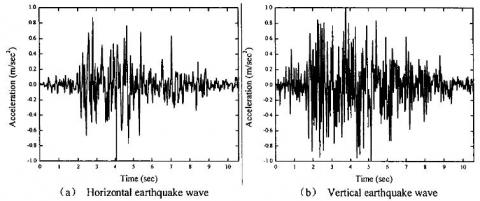Figure 9. The seismic wave of the target dam

Table 3. Extreme values of principal stresses

 Model 1 Model 2 Model 3 Maximum Principal Tensile Stress 7.52 2.72 2.61 Maximum Principal Stress 14.65 14.47 14.78

It can be seen from Table 3 that Model 1 had the highest maximum principal tensile stress. Compared with Models 2 and 3, the concrete strength in Model 1 increased while the tensile plastic strain decreased after considering the rate effect of concrete. The redistribution of principal tensile stress in the dam led to a certain variation in the principal stress. Despite the changes to the maximum value, the distribution of the maximum principal tensile stress remained the same.

The plastic strain rate had an obvious impact on the strength and elastic modulus of concrete. The three models outputted similar distributions of plastic strain rate, but differed in the magnitude. For Models 1 and 2, the maximum tensile plastic strain rates were $7.265×10^P{-2}$ and $8.176×10^{-3}$ , respectively, both of which appeared near the left abutment on the upstream surface. Under the multiaxial stress, Model 1 had a slightly lower yield strength than Model 2, and a greater plastic strain than the latter. The plastic strain rate of Model 1 was also significantly larger (4.1%) than that of Model 2.

6. Conclusion

The theoretical derivation of concrete constitutive model is a complex and difficult task. On the one hand, the established model should be able to simulate the exact deformation and strength features of concrete. On the other hand, the derivation process and the model should be simple and convenient. In this paper, a constitutive model of concrete is established based on thermodynamic principles and applied to the seismic analysis of an actual arch dam. The experimental results show that the proposed model is feasible, and that the tensile strength of concrete and the tensile stress of the dam can be improved after considering the effect of strain rate on concrete performance.

References

 Winnicki A, Pearce CJ, Bićanić N. (2001). Viscoplastic Hoffman consistency model for concrete. Computers & Structures 79(1): 7-19. https://doi.org/10.1016/s0045-7949(00)00110-3

 Liu HF, Liu HY, Song WD. (2010). Fracture characteristics of concrete subjected to impact loading. Science China 53(2): 253-261. https://doi.org/10.1007/s11433-009-0268-x

 Haido JH, Bakar BHA, Abdul-Razzak AA. (2011). Simulation of dynamic response for steel fibrous concrete members using new material modeling. Construction and Building Materials 25(3): 1407-1418. https://doi.org/10.1016/j.conbuildmat.2010.09.002

 Sun L, Zhu Y. (2013). A serial two-stage viscoelastic–viscoplastic constitutive model with thermodynamical consistency for characterizing time-dependent deformation behavior of asphalt concrete mixtures. Construction and Building Materials 40: 584-595. https://doi.org/10.1016/j.conbuildmat.2012.10.004

 Häussler-Combe U, Hartig J. (2008). Formulation and numerical implementation of a constitutive law for concrete with strain-based damage and plasticity. International Journal of Non-Linear Mechanics 43(5): 399-415. https://doi.org/10.1016/j.ijnonlinmec.2008.01.005

 Wu JY, Xu SL. (2011). An augmented multicrack elastoplastic damage model for tensile cracking. International Journal of Solids and Structures 48(18): 2511-2528. https://doi.org/10.1016/j.ijsolstr.2011.05.001

 Vignjevic R, Djordjevic N, Gemkow S. (2014). SPH as a nonlocal regularisation method: Solution for instabilities due to strain-softening. Computer Methods in Applied Mechanics and Engineering 277: 281-304. https://doi.org/10.1016/j.cma.2014.04.010

 Cicekli U, Voyiadjis GZ, Al-Rub RKA. (2007). A plasticity and anisotropic damage model for plain concrete. International Journal of Plasticity 23(10-11): 1874-1900. https://doi.org/10.1016/j.ijplas.2007.03.006

 Hameed R, Sellier A, Turatsinze A. (2011). Damage model for concrete reinforced with sliding metallic fibers. International Journal of Mechanics & Materials in Design 7(1): 83-97. https://doi.org/10.1007/s10999-011-9152-8

 Shang HS, Song YP, Qin LK. (2008). Experimental study on strength and deformation of plain concrete under triaxial compression after freeze-thaw cycles. Building & Environment 43(7): 1197-1204. https://doi.org/10.1016/j.buildenv.2006.08.027

 Lim JC, Ozbakkaloglu T. (2014). Stress–strain model for normal- and light-weight concretes under uniaxial and triaxial compression. Construction and Building Materials 71: 492-509. https://doi.org/10.1016/j.conbuildmat.2014.08.050

 Ispir M, Ilki A. (2013). Behavior of Historical Unreinforced Brick Masonry Walls under Monotonic and Cyclic Compression. Arabian Journal for Science & Engineering 38(8): 1993-2007. https://doi.org/10.1007/s13369-013-0567-4

 Aref AJ, Dolatshahi KM. (2013). A three-dimensional cyclic meso-scale numerical procedure for simulation of unreinforced masonry structures. Computers & Structures 120(2): 9-23. https://doi.org/10.1016/j.compstruc.2013.01.012

 Sfer D, Carol I, Gettu R. (2002). Study of the behavior of concrete under triaxial compression. Journal of Engineering Mechanics 128(2): 156-163. https://doi.org/10.1061/(ASCE)0733-9399(2002)128:2(156)

 Huang Y, Li J, Teng Y. (2017). Numerical simulation study on macroscopic mechanical behaviors and micro-motion characteristics of gangues under triaxial compression. Powder Technology 320: 668-684. https://doi.org/10.1007/s00603-011-0195-9

 Amann F, Kaiser P, Button EA. (2012). Experimental study of brittle behavior of clay shale in rapid triaxial compression. Rock Mechanics and Rock Engineering 45(1): 21-33. https://doi.org/10.1007/s00603-011-0195-9

 Samani AK, Attard MM. (2012). A stress–strain model for uniaxial and confined concrete under compression. Engineering Structures 41: 335-349. https://doi.org/10.1016/j.engstruct.2012.03.027

 Lim JC, Ozbakkaloglu T. (2014). Stress–strain model for normal- and light-weight concretes under uniaxial and triaxial compression. Construction and Building Materials 71: 492-509. https://doi.org/10.1016/j.conbuildmat.2014.08.050

 Wu JY, Xu SL. (2011). An augmented multicrack elastoplastic damage model for tensile cracking. International Journal of Solids and Structures 48(18): 2511-2528. https://doi.org/10.1016/j.ijsolstr.2011.05.001

 Fathima KMP, Kishen JMC. (2015). A thermodynamic correlation between damage and fracture as applied to concrete fatigue. Engineering Fracture Mechanics 146: 1-20. https://doi.org/10.1016/j.engfracmech.2015.07.019

 Zhu WC, Tang CA. (2002). Numerical simulation on shear fracture process of concrete using mesoscopic mechanical model. Construction & Building Materials 16(8): 453-463. https://doi.org/10.1016/s0950-0618(02)00096-x

 Ma C, Chen WZ, Sun JY. (2016). Numerical implementation of spatial elastoplastic damage model of concrete in the framework of isogeometric analysis Approach. Mathematical Problems in Engineering (10): 1-13. https://doi.org/10.1155/2016/4273024

 Yu H, Tang M, Wu J. (2011). Research on capacity of beam based on damage constitutive model for concrete. Chinese Journal of Applied Mechanics 28(4): 388-231. https://doi.org/10.1631/jzus.B1000185

 Bazant ZP, Bhat PD. (1976). Endochronic theory of inelasticity and failure of concrete. ASCE J Eng Mech Div 102(4): 701-722. https://doi.org/10.1016/0022-460X(76)90098-5

 Dell'Isola F, Sciarra G, Vidoli S. (2009). Generalized Hooke's law for isotropic second gradient materials. Proceedings Mathematical Physical & Engineering Sciences 465(2107): 2177-2196. https://doi.org/10.1098/rspa.2008.0530